﻿ The Manometer Is The Device For Measurement Of Pressure. Manomet Stock Photo - Image of engineering, background: 135495088

The manometer is the device for measurement of pressure. Manomet. Engineering, background.

The manometer is the device for measurement of pressure

More similar stock images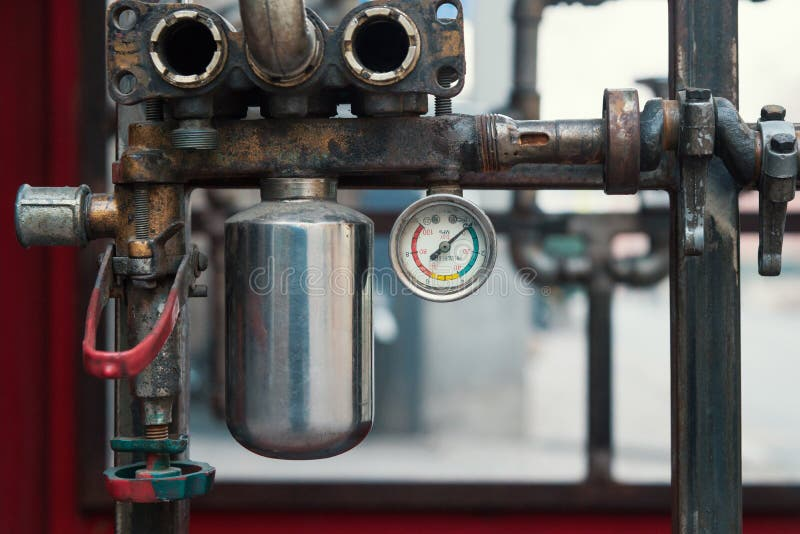manometer and Pressure device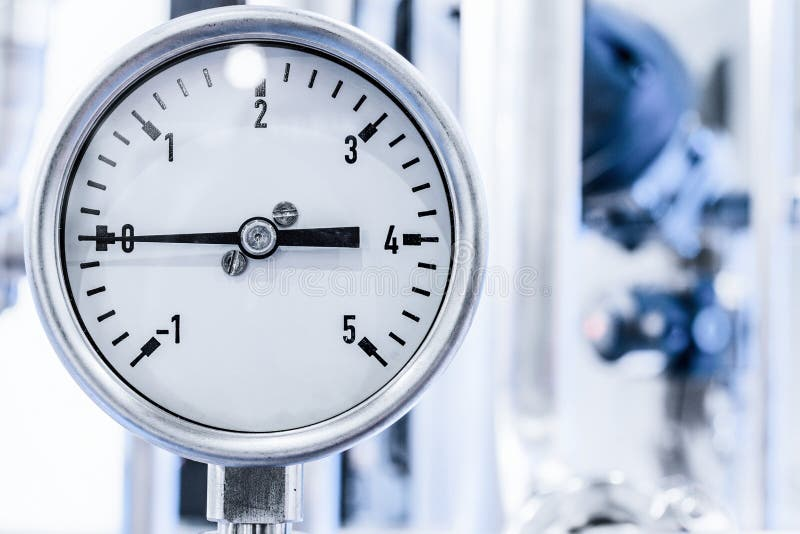The measuring device is a manometer.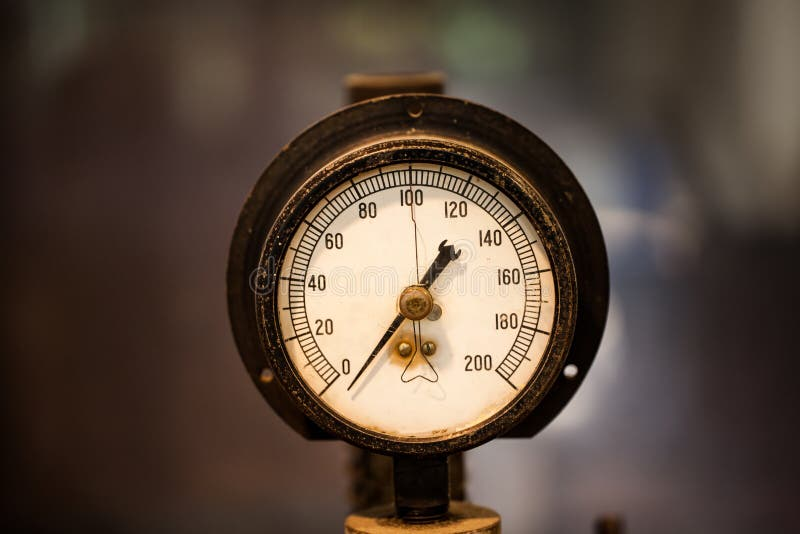Dial of manometer mechanical device in laboratory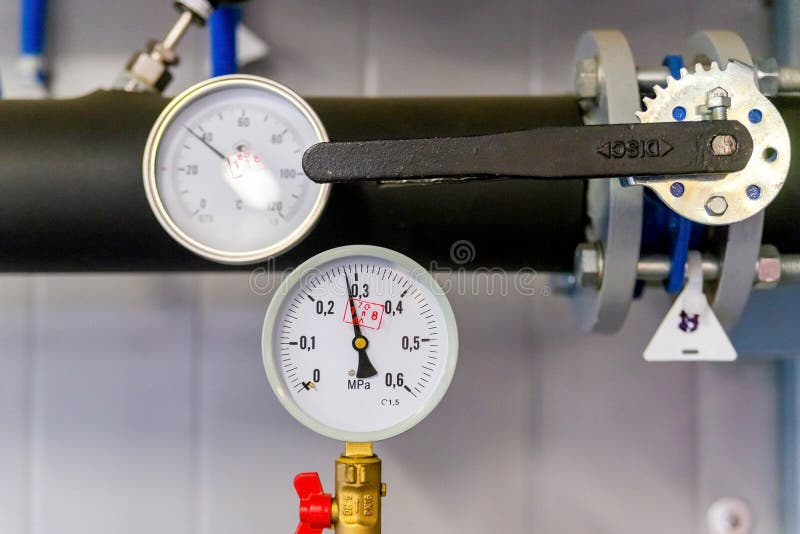Closeup of manometer, measuring gas pressure. Pipes and valves at industrial plant. Pressure gauge, measuring instrument close up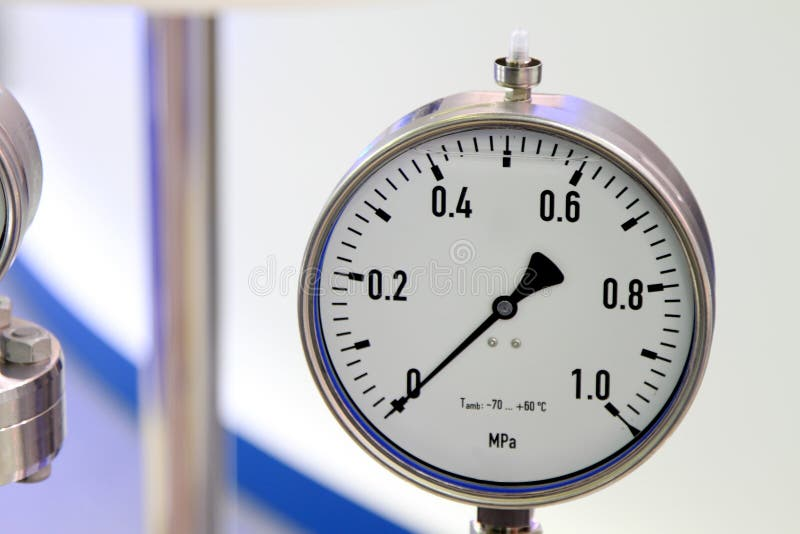Manometer - a device for measuring the pressure of fluid in the pipeline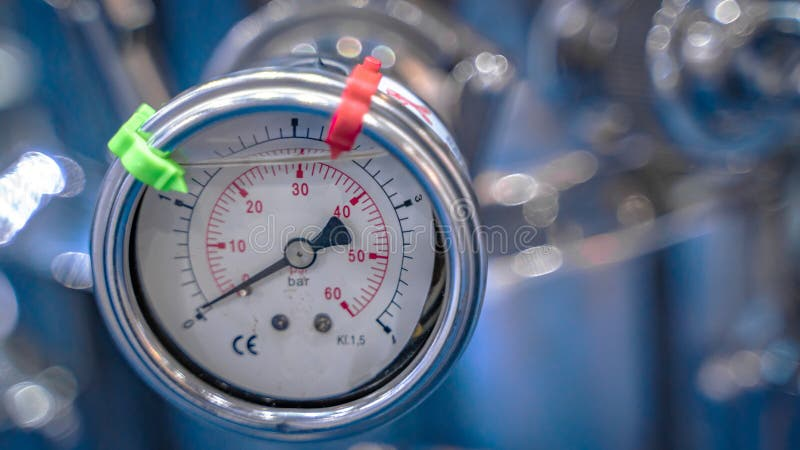Device For Measuring Absolute Pressure Gauge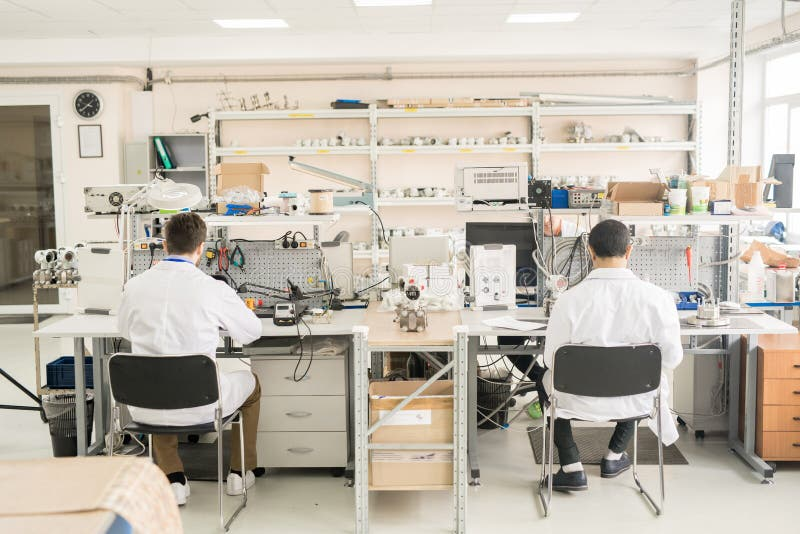Workplace of manometer plant staff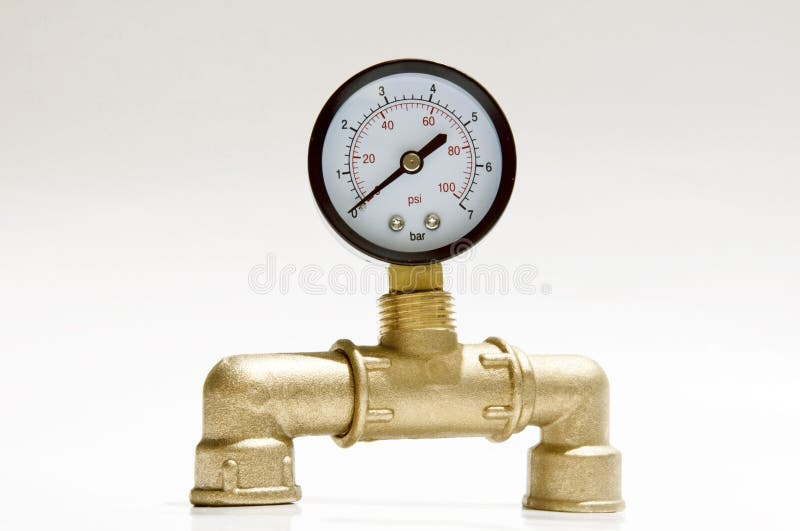Manometer on a white background, device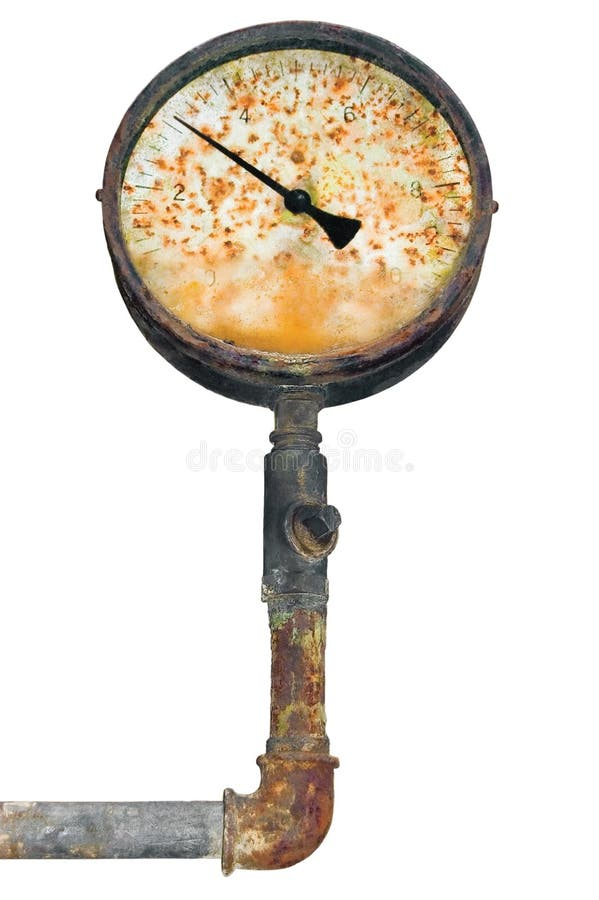Industrial manometer, isolated pressure gauge, ruined rust meter
More stock photos from Fotogigi85's portfolio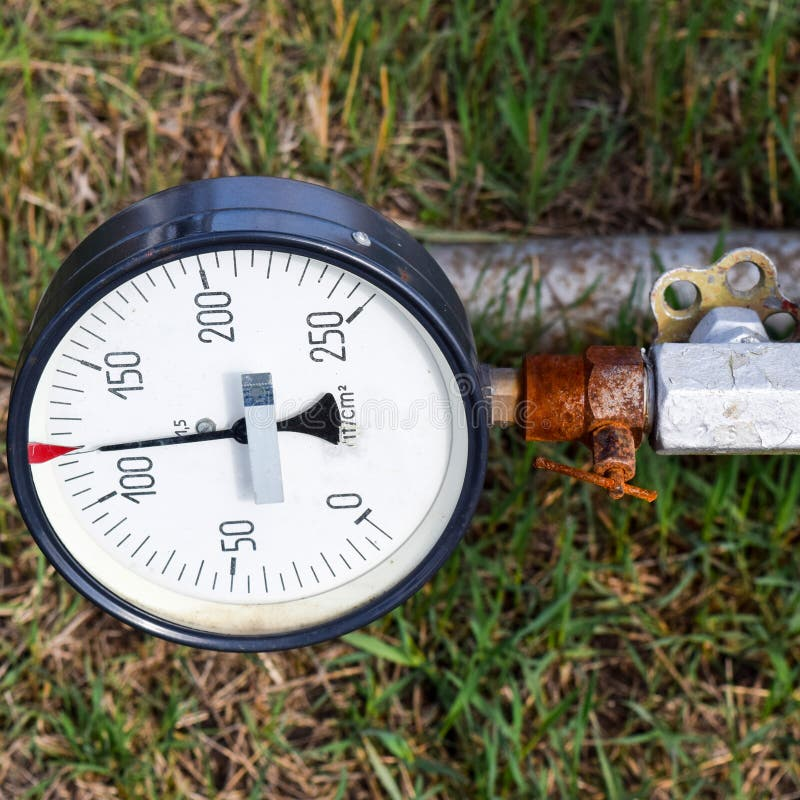The manometer is the device for measurement of pressure. Manomet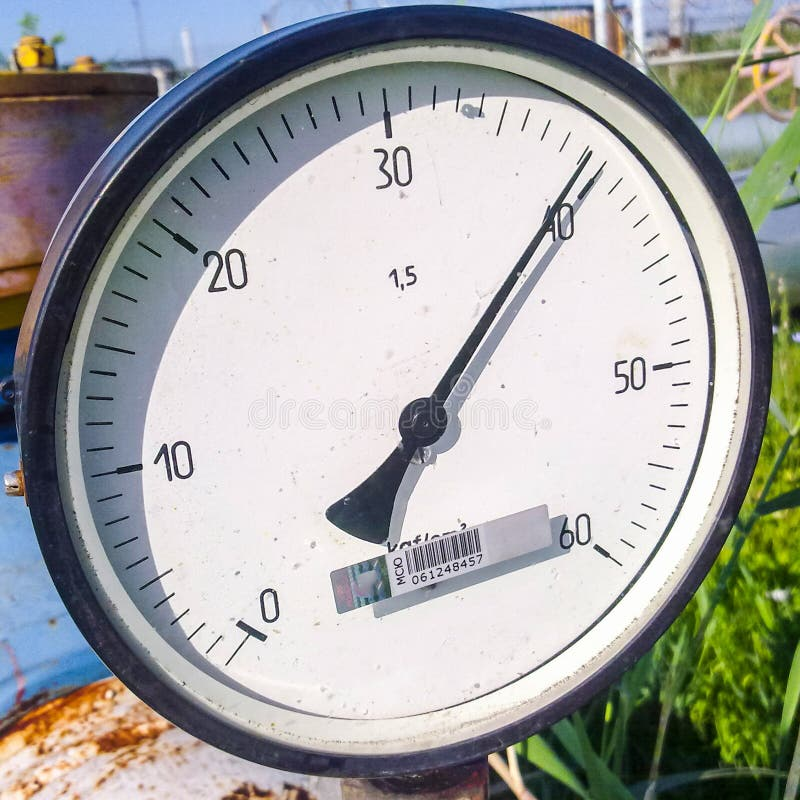The manometer is the device for measurement of pressure. Manomet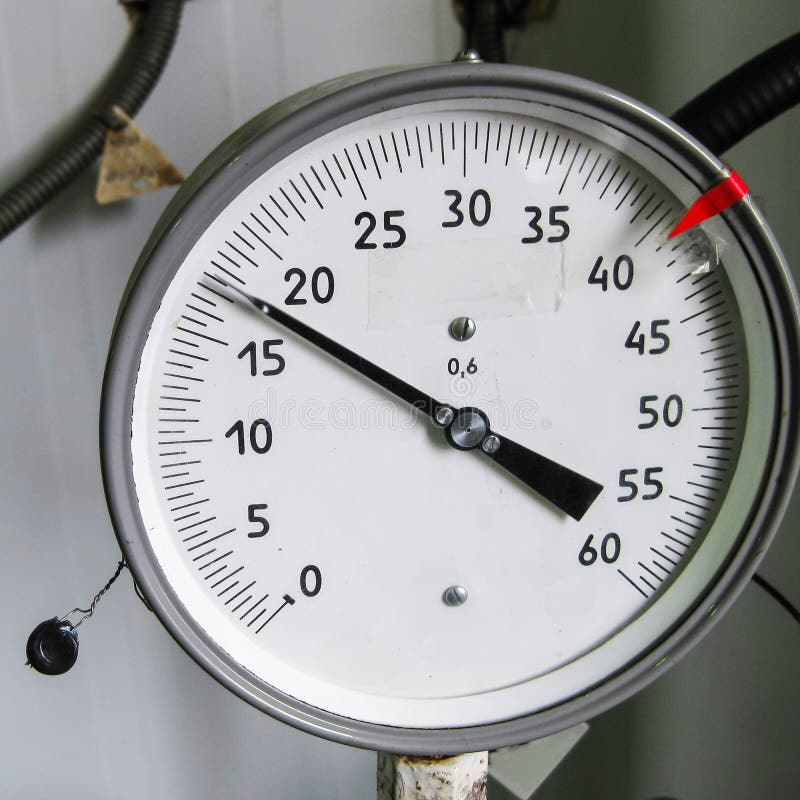The manometer is the device for measurement of pressure. ManometThe manometer is the device for measurement of pressure. Manomet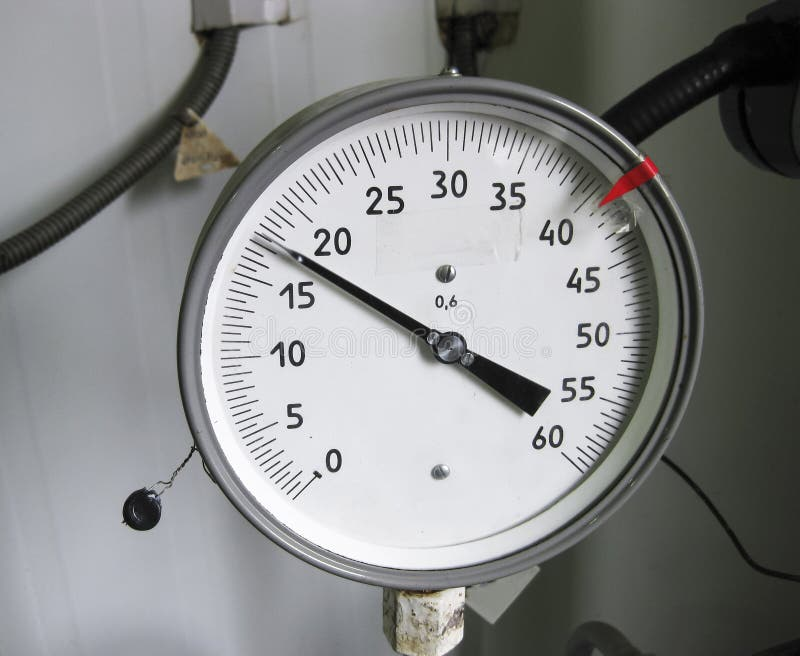The manometer is the device for measurement of pressure. Manomet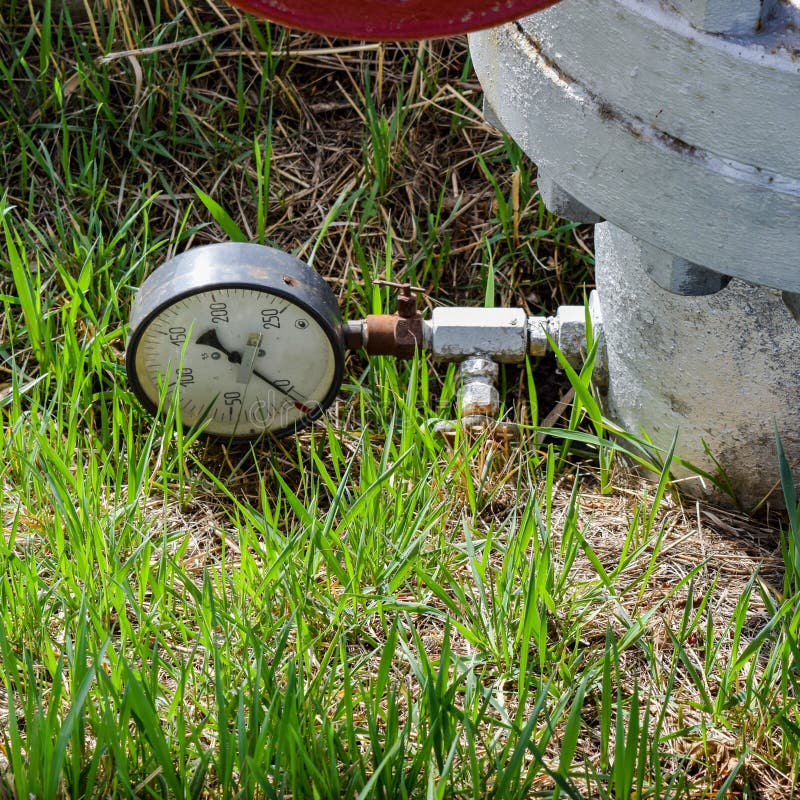The manometer is the device for measurement of pressure. Manomet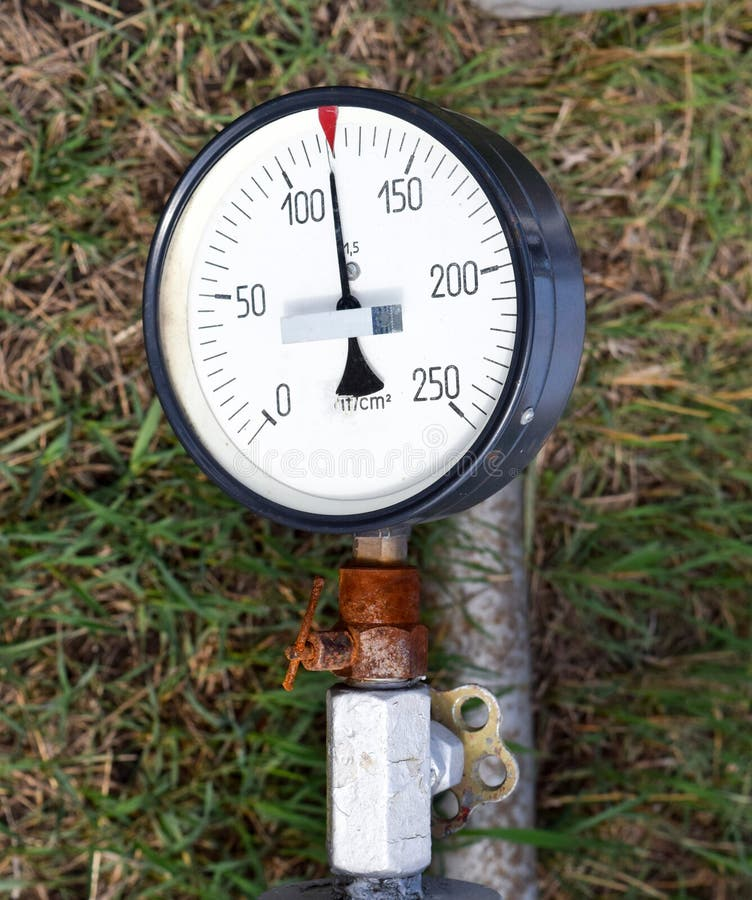The manometer is the device for measurement of pressure. Manomet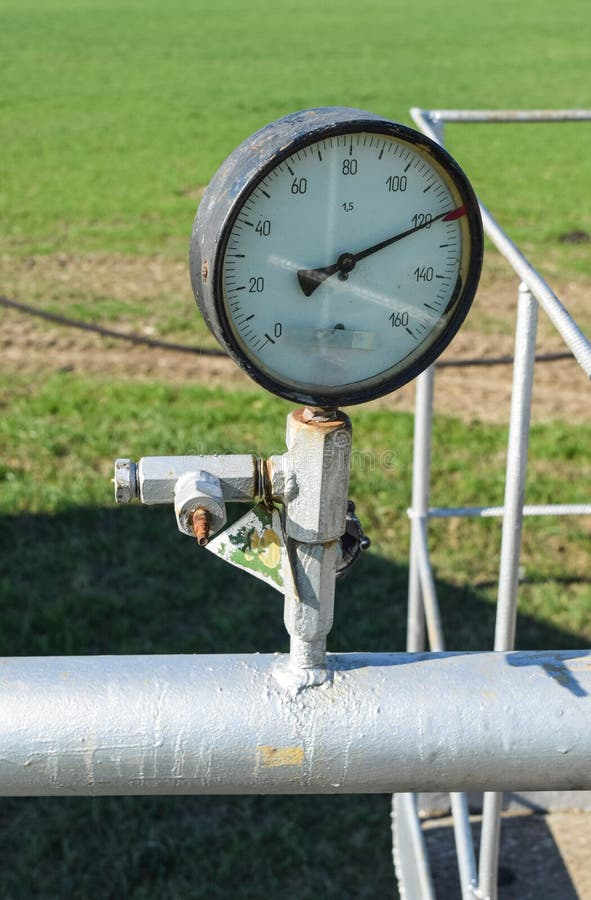The manometer is the device for measurement of pressure. Manometer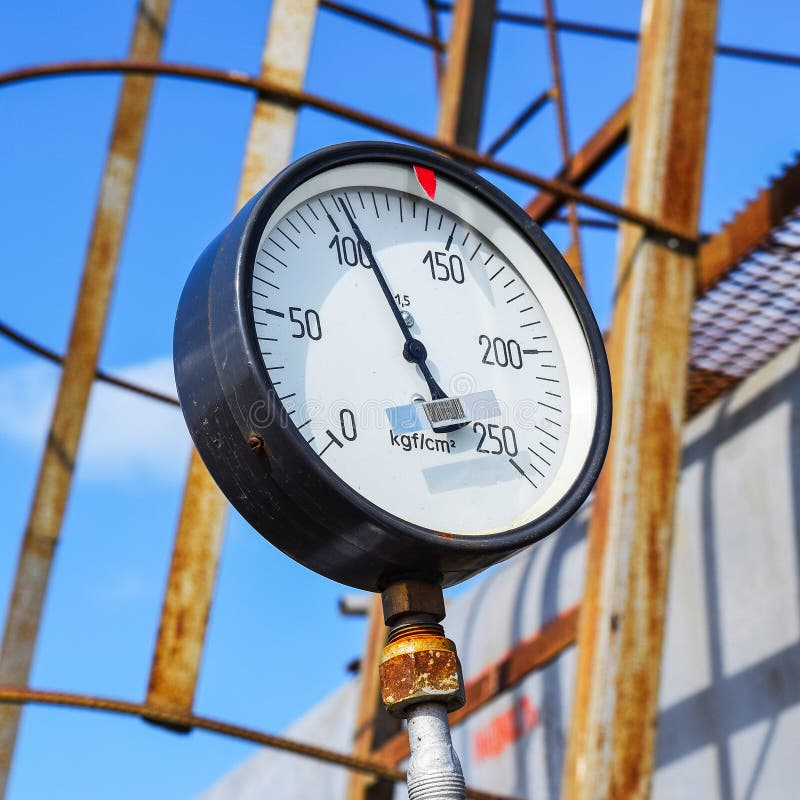The manometer is the device for measurement of pressure. Manomet